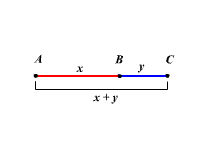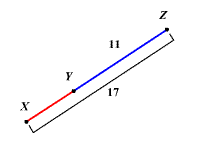The segment addition postulate is a fairly obvious-seeming postulate which states that:

If a point $B$ lies on a line segment $\stackrel{¯}{AC}$ , then $AB+BC=AC$ .

That is, the distance from one endpoint to the other is the sum of the distances from the middle point to either endpoint.Example:

Find the length $XY$ in the figure shown.By the Segment Addition Postulate, $XY+YZ=XZ$ .

Substitute the given values.

$XY+11=17$

Solve for $XY$ .

$XY=6$